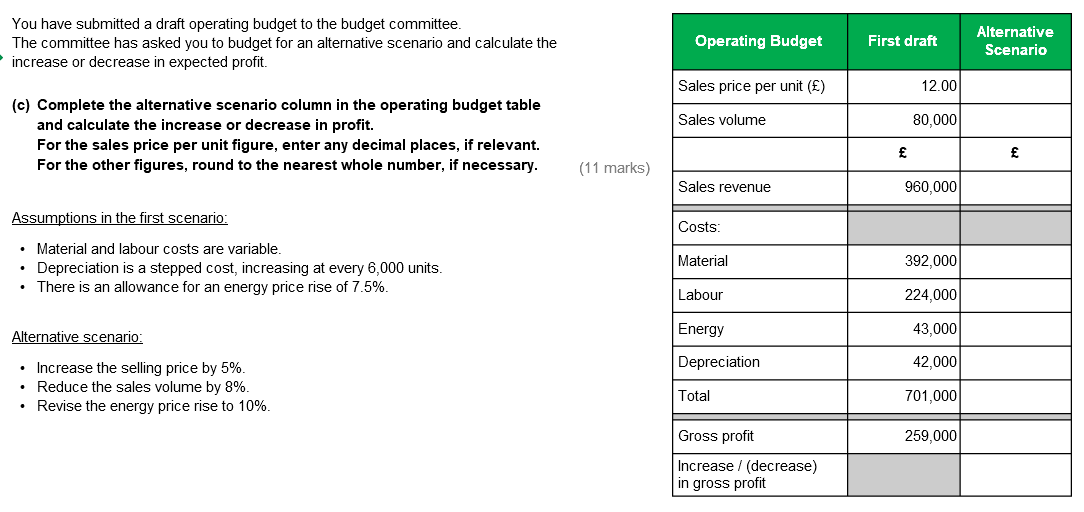Hi,
Can someone please help me calculate the energy cost for the alternative scenario?• Hi Hazel,

These are my workings:

Selling price per unit £12 * 1.05 (increase by 5%) = £12.60
Sales volume: 80,000 * 0.92 (8% decrease) = 73,600
Sales revenue: 73600 * 12.6 = 927,360
Material: 392000/80000 = 4.9, then * 73600 = 360,640
Labour: 224000/80000 = 2.8, then *73600 = 206,080
Energy: £43,000 = 107.5%. So to get 100%: (7.5/107.5) x 43000 = £40k. Then *1.1 = £44k.
Depreciation: 80,000/6000 = 13.3 , so 14 steps.
New sales volume of 73,600 / 6000 = 12.26, so 13 steps.
13 steps * (£42,000/14) = £39,000

Rest should be self explanatory.

Hope this helps

Regards
• In the first draft the energy cost is 100% + 7.5% alliwance, therefore
£43000/107.5=£400 per 1 %

We are then told that in the new draft the price rise will be 10%, so
110x400=£44000
AAT Level 2. 2018 Distinction
AAT Level 3. 2018 Distinction
AAT Level 4. 2019 FSLC 100% MABU 93% MADC 82% PDSY 80%